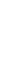a physical description of lift
extracts from www.rmcs.cranfield.ac.uk

There are several "definitions" of lift that may be found by searching through the relevant literature but only one that is really fundamentally correct. Sometimes the lift is defined as "the force that is required to support the weight of the aircraft in flight". On first inspection this seems to make sense and indeed it is true in the case of steady level flight. However, it is otherwise patently not the case, e.g. when the aircraft is performing a manoeuvre. The only truly correct definition which works for all possible flight conditions is:

the component of aerodynamic force generated on the aircraft which acts perpendicular to the instantaneous flight direction.

This means that the lift is inclined backwards in a climb, forwards in a dive and inwards in the case of a banked turn (Fig 1).Lift Force acting on an Aircraft

The overall force may be attributed to two natural phenomena:

• the variable static pressure distribution, acting perpendicular to the surface.
• the variable shear stress distribution, acting tangential to the surface.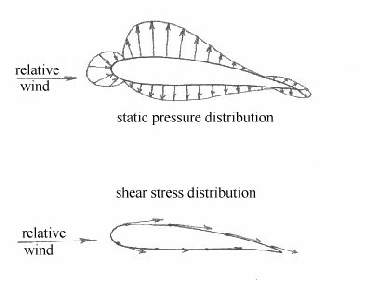Static Pressure & Shear Stress Distributions

• It is clear that, as far as lift is concerned, the shear stress is secondary and contributes mainly to the drag force. The lift force itself is primarily due to the pressure distribution imbalance over the upper and lower surfaces. In particular, the production of a lower net pressure across the surface relative to the lower surface will produce an upwards lift force. It is therefore often convenient to only deal with the pressure forces when discussing the physical origin of the lift force. This will form the basis of all the following reasoning on the subject.

### Aerofoil Section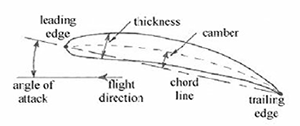Subsonic Aerofoil Section Geometry

Most of this discussion on lift will be centred on typical subsonic aerofoil sections. and it is therefore worthwhile reviewing their basic geometry. This is shown above, with the camber and thickness exaggerated to aid clarity (more typically around 3% and 12% of the chord length respectively). It is worth noting that any shape may be made to produce lift , provided it is given either camber or inclined to the flow direction (i.e. given an angle of attack). It is the need to optimise the lift/drag ratio which leads to the use of the classical streamlined aerofoil sections.

### Magnus Effect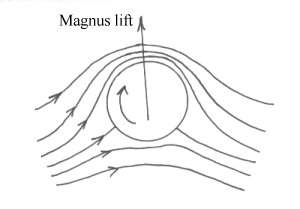From the discussion on the circulation theory of lift it should be apparent that any rotating object placed in a moving air stream will produce circulation and hence aerodynamic lift. This is then known as the magnus lift and produces many familiar effects such as the top/back/side spin on tennis balls and the hook/slice of a golf ball.

### Normal Force & Lift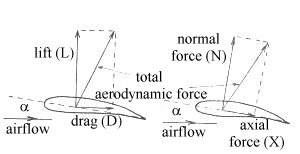Lift/Drag and Normal Force/Axial Force

While it is mostly convenient to deal with aerodynamic lift, there are occasions when it is best to make use of a different resolution of the aerodynamic force in which it is broken down into normal force and axial force instead of lift and drag. It should be recalled that lift and drag are the resolved components relative to the airflow direction (lift is perpendicular to it, drag is parallel to it). If the total force is resolved relative to the chord line of the wing instead, then the components are known as the normal force and axial force. The vector sum of the two components will always be the same, of course, i.e. equal to the total aerodynamic force.

A pair of general trigonometric relationships between the components may easily be derived:

L = N cosα - X sinα

D = N sinα + X cosα

Clearly, in cases where the chord line and airflow are coincident (i.e. when α = 0), then L = N and D = X. The use of N instead of L is especially useful when it comes to dealing with the stability and control aspects of an aircraft.

### Lift Curves of Finite Wings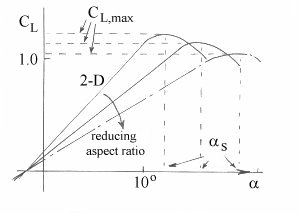Aspect Ratio Effect Upon Lift Curve Slope

The lift curve slope (a) reduces as aspect ratio reduces, as shown in Fig 1. This is due to the downwash angle produced, which reduces the effective angle of attack of the wing . A useful expression for determining the lift curve slope for an unswept finite wing is:

a = ao / [(1 + 57.3 ao) / (π e A)] per degree

where:

ao is the 2-D lift curve slope,

e is the span effectiveness (Oswald) factor, and

A is the wing aspect ratio.

The aspect ratio is the primary factor as both e and ao are reasonably similar for most conventional wing designs. The 2-D lift curve slope is obtained from specific aerofoil data and is theoretically equal to 2π per radian (or 0.1097 per degree) for a thin aerofoil but is more typically around 0.105 per degree. The curves are also slightly affected by Reynolds number (Re) and the application of high lift devices.

### Example

Calculate the lift curve slope for a NACA 23012 wing of 2-D lift curve slope = 0.106 per degree, aspect ratio = 10, Re = 5 x 106 and span effectiveness factor = 0.95.

Solution:

a = ao / [(1 + 57.3 ao) / (π e A)] = 0.088 per degree

### Wing Sweep and Mach Number Effects

Both wing sweep (Λ¼) and Mach number (M) affect the lift curve slope. A useful expression for its derivation (ref Howe) in this instance is given below:

a = 2π / [(0.32 + (0.16 A / cos Λ¼)) (1 - (M cosΛ¼)2)½] per radian

Note that the Oswald efficiency factor and 2-D lift curve slope terms are no longer present as it is the sweep, aspect ratio and Mach number which are the dominant factors.

### Example

Calculate the lift curve slope for a wing of aspect ratio = 10, quarter-chord sweepback angle = 33o and M = 0.82.

Solution: a = 2π / [(0.32 + (0.16 A / cos Λ¼)) (1 - (M cosΛ¼)2)½] = 2π / 1.617 = 3.88 per radian or 0.068 per degree

### Alternative Lift Theory - Newton's 3rd Law of Motion

An alternative explanation which is sometimes given in textbooks on flight mechanics uses Newton's laws of motion as a basis. The reasoning is that the wing deflects the air downwards so that the wing imparts a downward component to it. To do so, the wing must be providing a downwards force on the air (Newton's 1st Law). Then from Newton's 3rd Law, the air must be providing an equal and opposite reaction force on the wing.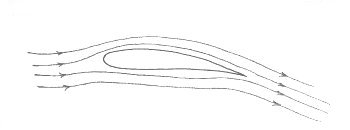Downwards Air Deflection over a Lifting Wing

This explanation is certainly better than the standard but it could be argued that this is the effect of lift rather than the cause of it. There is no doubt that air is deflected downwards and indeed that a considerable amount of air movement is required in order to provide the requisite amount of wing lift. It could, however, be the pressure distribution which is pushing the wing up and that this is really the action which is taking place. The reaction to this would then be for the wing to push the air down, with a sufficient momentum change to balance out the lift produced. This would mean that the original argument is probably the "wrong way around"!

### Drag Components - Trailing Vortex Drag

Any lift-producing surface, such as an aircraft wing, will inevitably produce compensating wing-tip vortices. This is due to the presence of a relatively low pressure on the wing upper surface compared with the lower surface. This pressure difference must equalise at the wing tips and the net result is a movement of airflow along the span of the wing, from the high pressure region to the low pressure region. The airflow therefore tends to move outwards towards the tip on the lower surface and inwards to the centre of the aircraft along the upper surface. When combined with the airstream this produces a pair of contra-rotating vortices at the wing tips.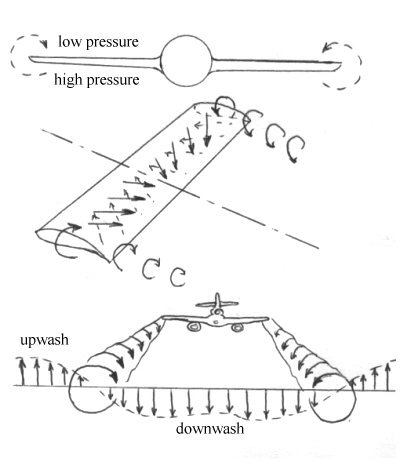Trailing Vortices Producing Downwash

The vortices force the air to move downwards across the whole span of the wing, known as downwash. Outboard of the wing tips, the air experiences an upwash flow, which explains why flocks of birds tend to fly in a V-formation.

The downwash component produces a drag component known as either trailing vortex drag or lift-induced drag, as shown below. The downwash component may be added to the original airstream velocity vector to produce a revised airflow component which is now at a lower angle of attack than previously. Since lift is proportional to angle of attack this means that the overall lift is reduced. It also means that the lift vector is tilted rearwards as it is defined as being perpendicular to the airstream vector. If the revised lift term is now split back into its original directional components it is clear that there must be a drag term (Di) present.Downwash Effect

The aspect ratio is the primary factor as both e and ao are reasonably similar for most conventional wing designs. The 2-D lift curve slope is obtained from specific aerofoil data and is theoretically equal to 2π per radian (or 0.1097 per degree) for a thin aerofoil but is more typically around 0.105 per degree. The curves are also slightly affected by Reynolds number (Re) and the application of high lift devices.

### Example

Calculate the lift curve slope for a NACA 23012 wing of 2-D lift curve slope = 0.106 per degree, aspect ratio = 10, Re = 5 x 106 and span effectiveness factor = 0.95.

Solution:

a = ao / [(1 + 57.3 ao) / (π e A)] = 0.088 per degree

### Wing Sweep and Mach Number Effects

Both wing sweep (Λ¼) and Mach number (M) affect the lift curve slope. A useful expression for its derivation (ref Howe) in this instance is given below:

a = 2π / [(0.32 + (0.16 A / cos Λ¼)) (1 - (M cosΛ¼)2)½] per radian

Note that the Oswald efficiency factor and 2-D lift curve slope terms are no longer present as it is the sweep, aspect ratio and Mach number which are the dominant factors.

### Example

Calculate the lift curve slope for a wing of aspect ratio = 10, quarter-chord sweepback angle = 33o and M = 0.82.

Solution: a = 2π / [(0.32 + (0.16 A / cos Λ¼)) (1 - (M cosΛ¼)2)½] = 2π / 1.617 = 3.88 per radian or 0.068 per degree

### Correct Physical Explanation of Origin of Lift

A more fundamentally correct explanation for the origin of aerodynamic lift may be found by making use of the conservation laws of physics, namely mass flow (continuity) and energy (Euler or Bernoulli).

Consider the 2-D stream tubes A and B shown below - these are originally of the same width when well upstream of the aerofoil and out of its influence. The common streamline between them is aligned with the leading edge stagnation point so acts to divide the airflow over the top and bottom surfaces. The stream tube A encounters the upper portion of the aerofoil and is "squashed" through what is effectively a smaller area. Stream tube B is squashed less resulting in a larger stream tube width relative to A. From continuity (dm/dt = ρ A V) this must mean that the velocity in A must be greater than in B.

Either Euler's (dp = - ρ VdV) or Bernoulli's (p + ½ ρ V2 = constant) theorems may now be applied to show that pA must be less than pB. This results in the pressure distribution shown below. It can be seen that the majority of lift is due to the low pressure (suction) acting over the upper surface, particularly over the front 20% to 30%, where the flow accelerations are at their most severe.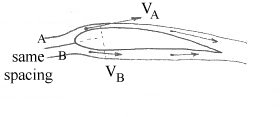Changes in Stream Tube Spacing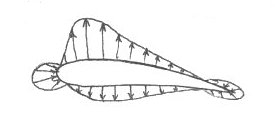Resultant Pressure Distribution

t) and the table puts an equal and opposite force on the object to hold it up. In order to generate lift a wing must do something to the air. What the wing does to the air is the action while lift is the reaction.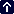Your browser does not support inline frames or is currently configured not to display inline frames.